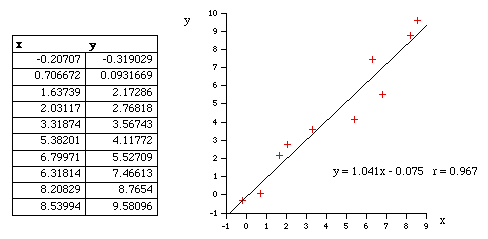Linear Regression
Windows only

## Overview

Linear regression is a method to best fit a linear equation, or a straight line, of the form $$y(x) = a + bx$$ to a collection of n points $$(x, y)$$, where $$b$$ is the slope and $$a$$ the intercept on the y-axis.

## Discussion

In the following implementation, the result will be stated below without derivation, that requires minimization of the sum of the squared distance from the data points and the proposed line. This function is minimized by calculating the derivative with respect to a and b and setting these to zero.

This method assumes there is no known variance for the x and y values. There are solutions which can take this into account, this is particularly important if some values are known with less error than others. Also, this method requires that the slope is not infinite…

' Description:
'   Linear Regression
'   y(x) = a + bx, for n samples.
' Parameters:
'   data - [in]  An array of (x,y) values.
'   a    - [out] The slope.
'   b    - [out] The y-axis intersect.
'   c    - [out] The regression coefficient.
' Returns:
'   True if successful, False otherwise.

Function LinearRegression(ByVal data, ByRef a, ByRef b, ByRef r)

' Local variables
Dim d, x, y, n
Dim sumx, sumy, sumx2, sumy2, sumxy, sxx, syy, sxy

' Initialize variables
sumx = 0 : sumy = 0 : sumx2 = 0 : sumy2 = 0 : sumxy = 0
n = UBound(data) + 1

' Initialize output
a = 0 : b = 0 : r = 0

' Default return value
LinearRegression = False

' Must have at least two points
If (n < 2) Then Exit Function

' Compute some things we need
For Each d In data
x = d(0)
y = d(1)
sumx  = sumx  + x
sumy  = sumy  + y
sumx2 = sumx2 + (x * x)
sumy2 = sumy2 + (y * y)
sumxy = sumxy + (x * y)
Next

sxx = sumx2 - (sumx * sumx / n)
syy = sumy2 - (sumy * sumy / n)
sxy = sumxy - (sumx * sumy / n)

' Infinite slope (b), non existant intercept (a)
If (Abs(sxx) = 0) Then Exit Function

' Compute slope (b) and intercept (a)
b = sxy / sxx
a = sumy / n - b * sumx / n

' Compute regression coefficient
If (Abs(syy) = 0) Then
r = 1
Else
r = sxy / Sqr(sxx * syy)
End If

LinearRegression = True

End Function


## Best FitThe following example shows the points and the best fit line as determined using the techniques demonstrated above…

Sub Main()

Dim data(9), a, b, r

data(0) = Array(-0.20707, -0.319029)
data(1) = Array(0.706672, 0.0931669)
data(2) = Array(1.63739, 2.17286)
data(3) = Array(2.03117, 2.76818)
data(4) = Array(3.31874, 3.56743)
data(5) = Array(5.38201, 4.11772)
data(6) = Array(6.79971, 5.52709)
data(7) = Array(6.31814, 7.46613)
data(8) = Array(8.20829, 8.7654)
data(9) = Array(8.53994, 9.58096)

If (LinearRegression(data, a, b, r) = True) Then
Call Rhino.Print("Slope (b) = " & FormatNumber(b, 3))
Call Rhino.Print("Y Intercept (a) = " & FormatNumber(a, 3))
Call Rhino.Print("Regression Coefficient = " & FormatNumber(r, 3))
End If

End Sub